# RD Sharma Solutions for Class 11 Chapter 32 - Statistics Exercise 32.3

This exercise mainly discusses mean deviation of a grouped or continuous frequency distribution. To help students score well in the board exams, experts at BYJU’S have solved the exercise wise problems using shortcut methods. They can solve these problems without any time constraint. These solutions help students clear their confusion about the concepts discussed here. Students can download the pdf of solutions based on their requirements. RD Sharma Class 11 Maths Solutions pdf is readily available, where students can download easily and start practising offline.

## Download the pdf of RD Sharma Solutions for Class 11 Maths Exercise 32.3 Chapter 32 – Statistics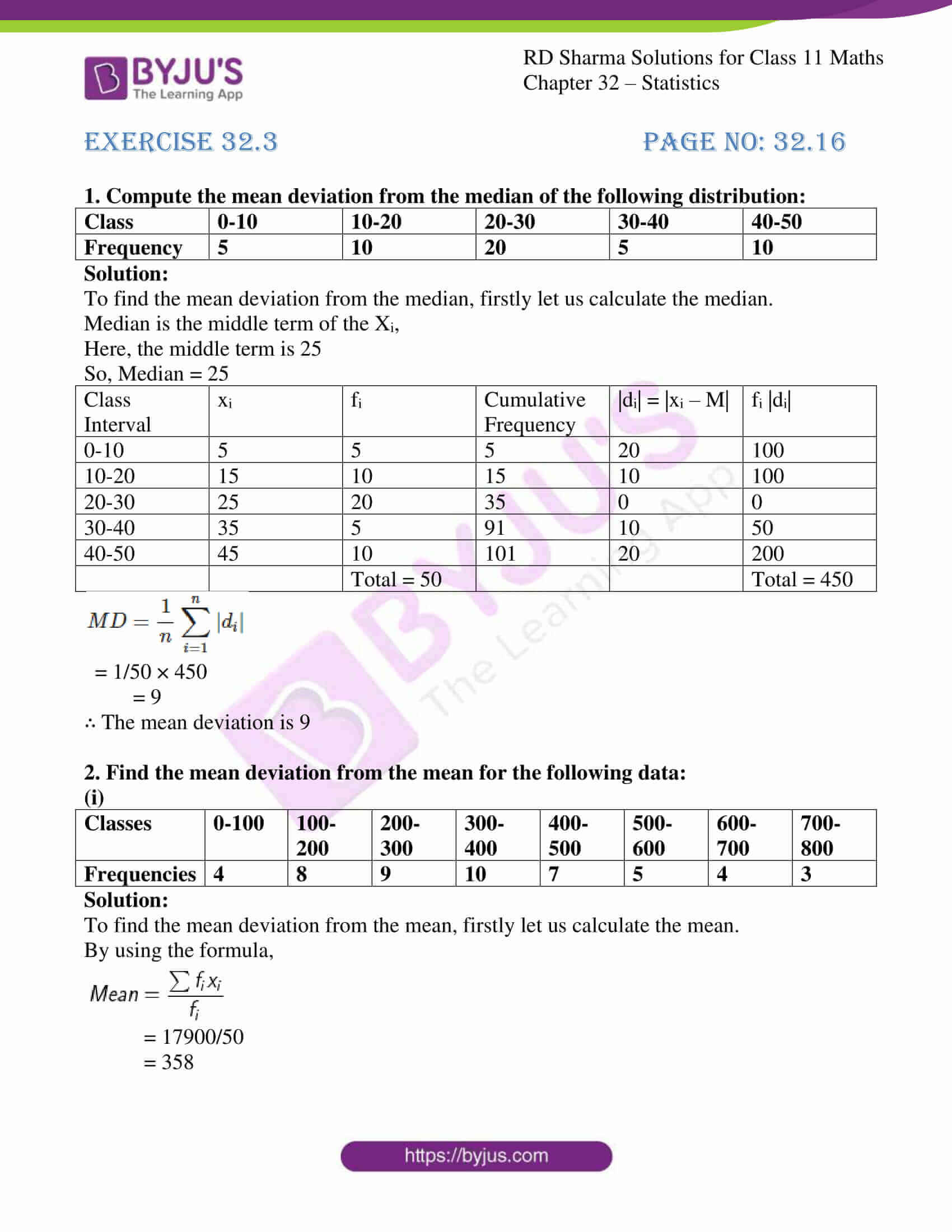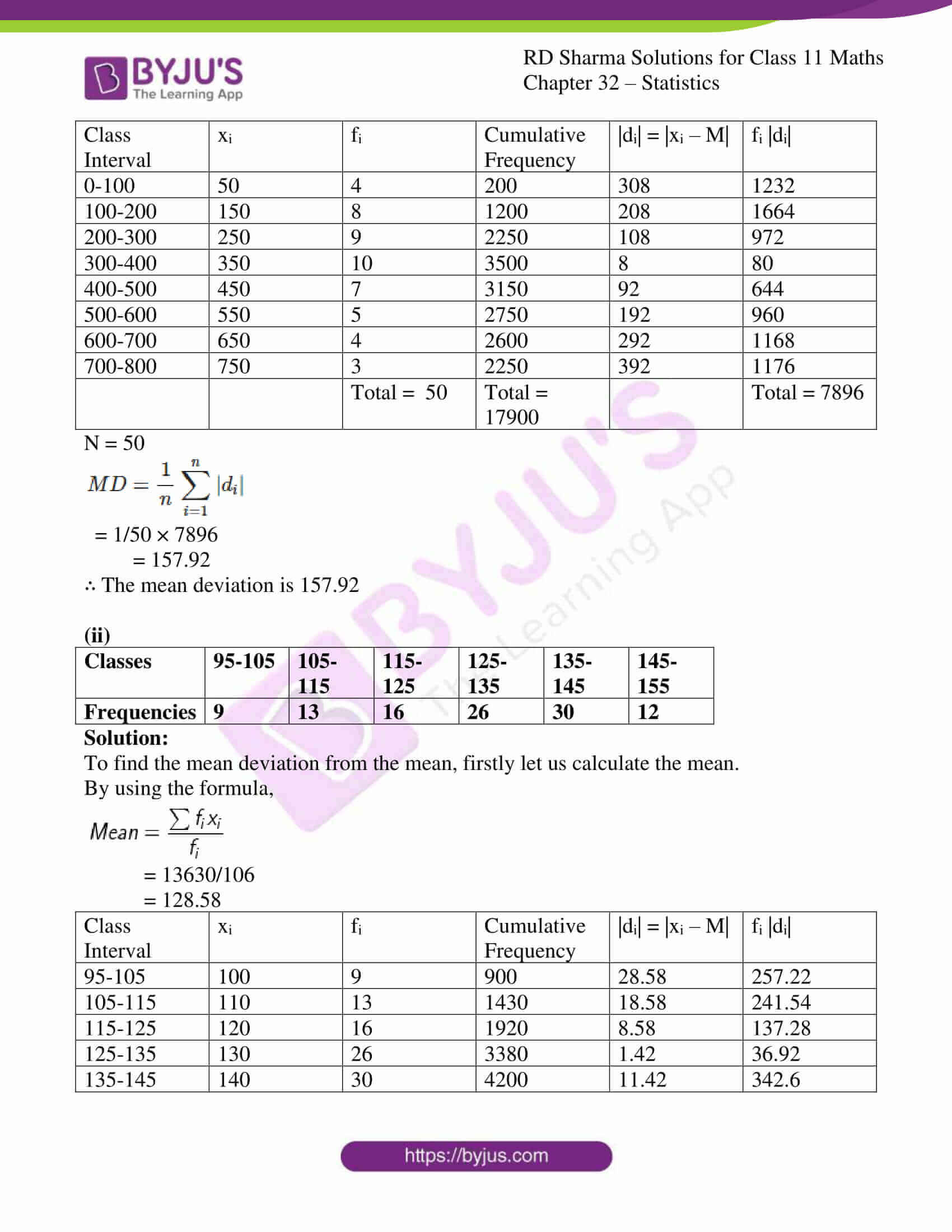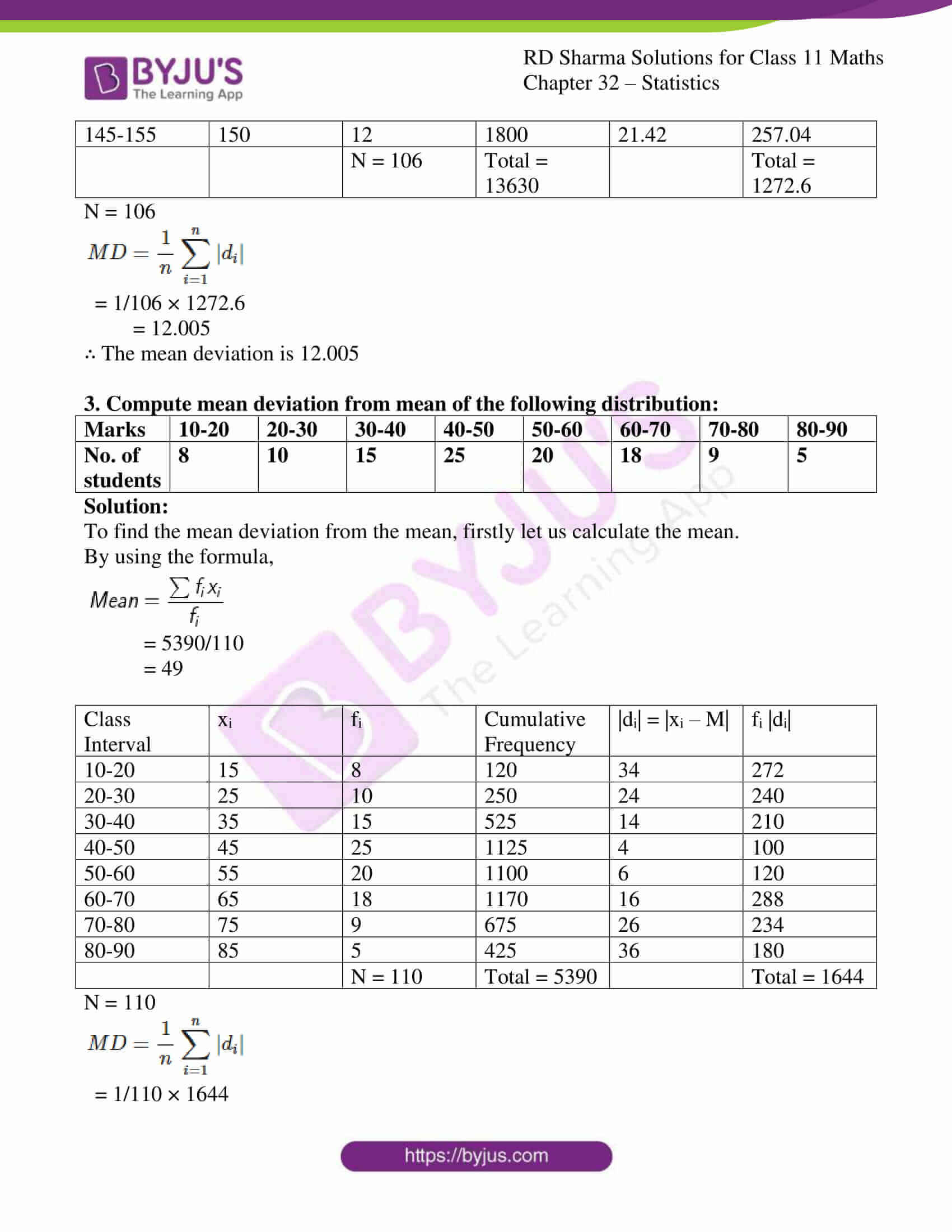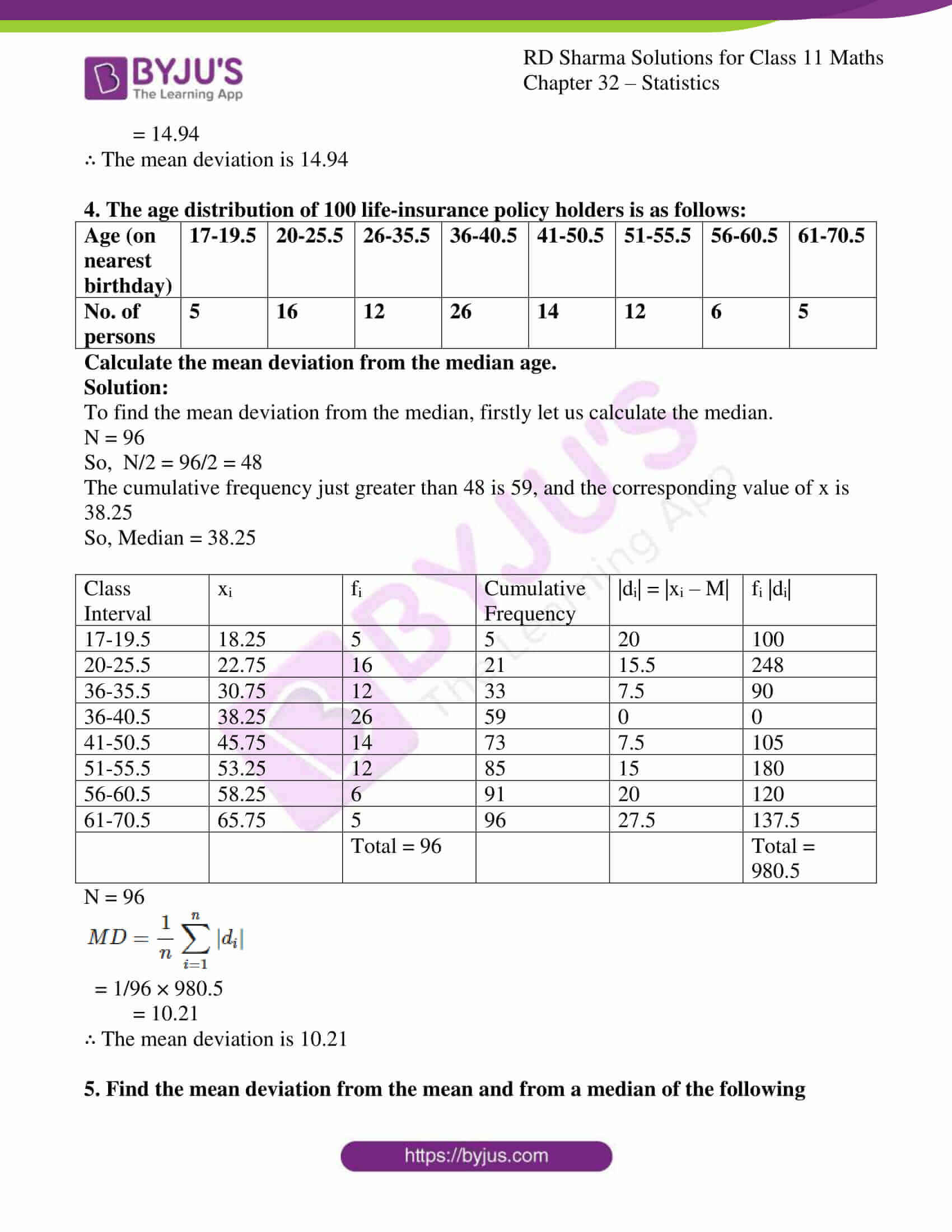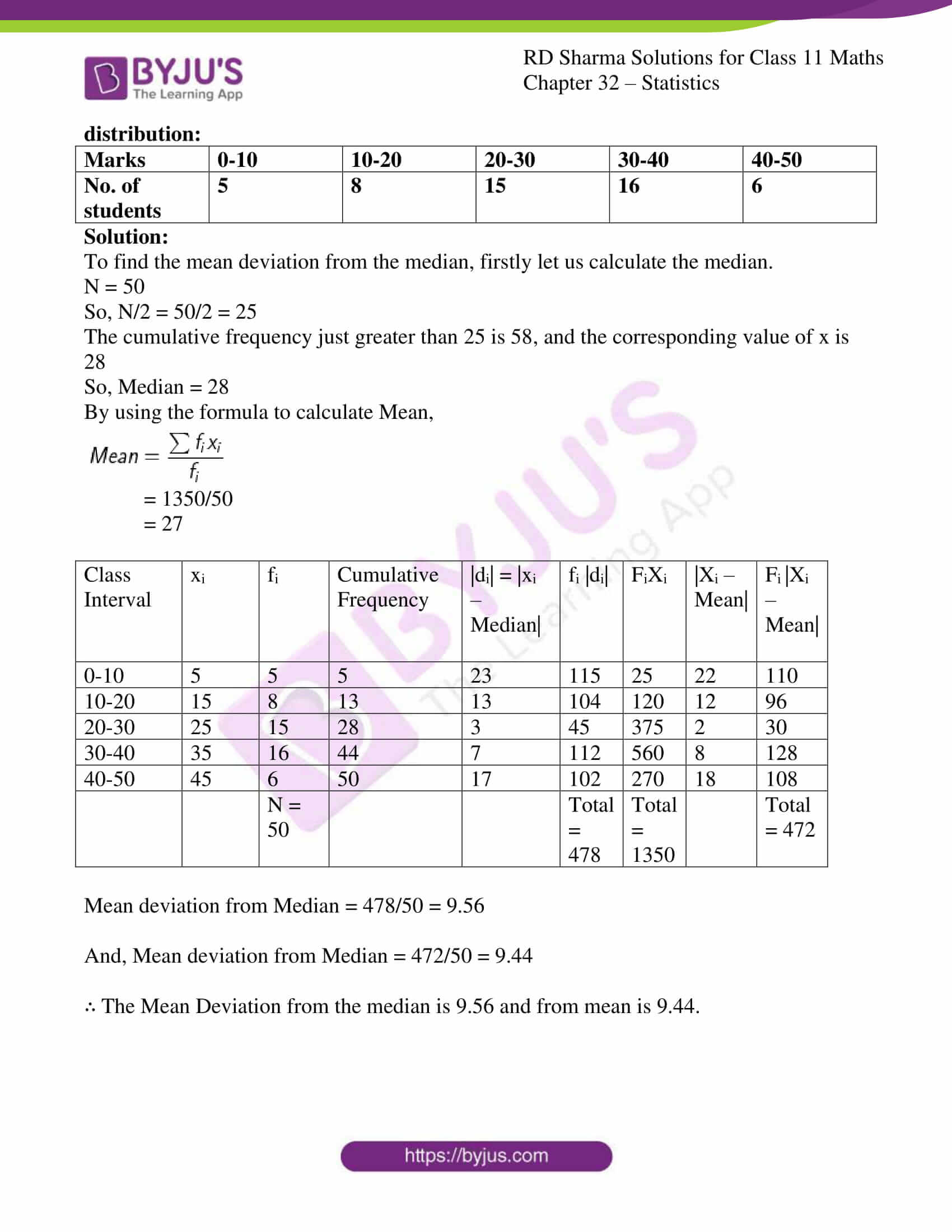### Access answers to RD Sharma Solutions for Class 11 Maths Exercise 32.3 Chapter 32 – Statistics

#### EXERCISE 32.3 PAGE NO: 32.16

1. Compute the mean deviation from the median of the following distribution:

 Class 0-10 10-20 20-30 30-40 40-50 Frequency 5 10 20 5 10

Solution:

To find the mean deviation from the median, firstly let us calculate the median.

Median is the middle term of the Xi,

Here, the middle term is 25

So, Median = 25

 Class Interval xi fi Cumulative Frequency |di| = |xi – M| fi |di| 0-10 5 5 5 20 100 10-20 15 10 15 10 100 20-30 25 20 35 0 0 30-40 35 5 91 10 50 40-50 45 10 101 20 200 Total = 50 Total = 450
$MD = \frac{1}{n}\sum_{i=1}^{n}|d_{i}|$

= 1/50 × 450

= 9

∴ The mean deviation is 9

2. Find the mean deviation from the mean for the following data:

(i)

 Classes 0-100 100-200 200-300 300-400 400-500 500-600 600-700 700-800 Frequencies 4 8 9 10 7 5 4 3

Solution:

To find the mean deviation from the mean, firstly let us calculate the mean.

By using the formula,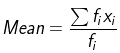= 17900/50

= 358

 Class Interval xi fi Cumulative Frequency |di| = |xi – M| fi |di| 0-100 50 4 200 308 1232 100-200 150 8 1200 208 1664 200-300 250 9 2250 108 972 300-400 350 10 3500 8 80 400-500 450 7 3150 92 644 500-600 550 5 2750 192 960 600-700 650 4 2600 292 1168 700-800 750 3 2250 392 1176 Total = 50 Total = 17900 Total = 7896

N = 50

$MD = \frac{1}{n}\sum_{i=1}^{n}|d_{i}|$

= 1/50 × 7896

= 157.92

∴ The mean deviation is 157.92

(ii)

 Classes 95-105 105-115 115-125 125-135 135-145 145-155 Frequencies 9 13 16 26 30 12

Solution:

To find the mean deviation from the mean, firstly let us calculate the mean.

By using the formula,= 13630/106

= 128.58

 Class Interval xi fi Cumulative Frequency |di| = |xi – M| fi |di| 95-105 100 9 900 28.58 257.22 105-115 110 13 1430 18.58 241.54 115-125 120 16 1920 8.58 137.28 125-135 130 26 3380 1.42 36.92 135-145 140 30 4200 11.42 342.6 145-155 150 12 1800 21.42 257.04 N = 106 Total = 13630 Total = 1272.6

N = 106

$MD = \frac{1}{n}\sum_{i=1}^{n}|d_{i}|$

= 1/106 × 1272.6

= 12.005

∴ The mean deviation is 12.005

3. Compute mean deviation from mean of the following distribution:

 Marks 10-20 20-30 30-40 40-50 50-60 60-70 70-80 80-90 No. of students 8 10 15 25 20 18 9 5

Solution:

To find the mean deviation from the mean, firstly let us calculate the mean.

By using the formula,= 5390/110

= 49

 Class Interval xi fi Cumulative Frequency |di| = |xi – M| fi |di| 10-20 15 8 120 34 272 20-30 25 10 250 24 240 30-40 35 15 525 14 210 40-50 45 25 1125 4 100 50-60 55 20 1100 6 120 60-70 65 18 1170 16 288 70-80 75 9 675 26 234 80-90 85 5 425 36 180 N = 110 Total = 5390 Total = 1644

N = 110

$MD = \frac{1}{n}\sum_{i=1}^{n}|d_{i}|$

= 1/110 × 1644

= 14.94

∴ The mean deviation is 14.94

4. The age distribution of 100 life-insurance policy holders is as follows:

 Age (on nearest birthday) 17-19.5 20-25.5 26-35.5 36-40.5 41-50.5 51-55.5 56-60.5 61-70.5 No. of persons 5 16 12 26 14 12 6 5

Calculate the mean deviation from the median age.

Solution:

To find the mean deviation from the median, firstly let us calculate the median.

N = 96

So, N/2 = 96/2 = 48

The cumulative frequency just greater than 48 is 59, and the corresponding value of x is 38.25

So, Median = 38.25

 Class Interval xi fi Cumulative Frequency |di| = |xi – M| fi |di| 17-19.5 18.25 5 5 20 100 20-25.5 22.75 16 21 15.5 248 36-35.5 30.75 12 33 7.5 90 36-40.5 38.25 26 59 0 0 41-50.5 45.75 14 73 7.5 105 51-55.5 53.25 12 85 15 180 56-60.5 58.25 6 91 20 120 61-70.5 65.75 5 96 27.5 137.5 Total = 96 Total = 980.5

N = 96

$MD = \frac{1}{n}\sum_{i=1}^{n}|d_{i}|$

= 1/96 × 980.5

= 10.21

∴ The mean deviation is 10.21

5. Find the mean deviation from the mean and from a median of the following distribution:

 Marks 0-10 10-20 20-30 30-40 40-50 No. of students 5 8 15 16 6

Solution:

To find the mean deviation from the median, firstly let us calculate the median.

N = 50

So, N/2 = 50/2 = 25

The cumulative frequency just greater than 25 is 58, and the corresponding value of x is 28

So, Median = 28

By using the formula to calculate Mean,= 1350/50

= 27

 Class Interval xi fi Cumulative Frequency |di| = |xi – Median| fi |di| FiXi |Xi – Mean| Fi |Xi – Mean| 0-10 5 5 5 23 115 25 22 110 10-20 15 8 13 13 104 120 12 96 20-30 25 15 28 3 45 375 2 30 30-40 35 16 44 7 112 560 8 128 40-50 45 6 50 17 102 270 18 108 N = 50 Total = 478 Total = 1350 Total = 472

Mean deviation from Median = 478/50 = 9.56

And, Mean deviation from Median = 472/50 = 9.44

∴ The Mean Deviation from the median is 9.56 and from mean is 9.44.